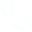# Python: Use of recursive and anonymous functions & file opening and closing

recursive function
<1>What is a recursive function
Through the previous study, we know that a function can call other functions.
If a function does not call other functions internally, but itself, the function is a recursive function.

<2> The function of recursive function
As an example, let's calculate the factorial n! = 1 2 3 ... n
Solution 1: Use a loop to complete

def cal(num):
result,i = 1,1
while i <= num:
result *= i
i+= 1
return result
print(cal(3))
Look at the law of factorial
1! = 1
2! = 2 × 1 = 2 × 1!
3! = 3 × 2 × 1 = 3 × 2!
4! = 4 × 3 × 2 × 1 = 4 × 3!
...
n! = n × (n-1)!
Solution 2: Use recursion to achieve
def factorial(num):
result = 1
if num == 1:
return 1
result = num * factorial(num -1)
return result
print(cal(3))

anonymous function
Small anonymous functions can be created using the lambda keyword. Such functions get their name from the omission of the standard step of declaring functions with def.
The syntax of a lambda function consists of only one statement, as follows:

lambda parameter list: operation expression
Examples are as follows:

sum = lambda arg1, arg2: arg1 + arg2
# call the sum function
print("Value of total : %d" % sum( 10, 20 ))
print("Value of total : %d" % sum( 20, 20 ))
The output of the above example:

Value of total: 30
Value of total: 40
Lambda functions can receive any number of parameters but can only return the value of one expression
Anonymous functions can execute arbitrary expressions (even print functions), but it is generally believed that expressions should have a calculation result for return.
Python can use lambda when writing some execution scripts, which can accept the process of defining functions, such as writing a simple script management server

Applications
function passed as parameter
>>> def fun(a, b, opt):
... print("a = " % a)
... print("b = " % b)
... print("result = "% opt(a, b))
...
a = 1
b = 2
result = 3

File opening and closing

If you want to write a resume in word, what should be the process?

1. Open the word software and create a new word file
2. Write personal resume information

3. Save the file
4. Close word software

Similarly, the overall process of operating files is very similar to the process of writing a resume in word

1. Open the file, or create a new file

3. Close the file

<1> Open the file
In python, use the open function to open an existing file or create a new file
Open parameter introduction

file: used to specify the open file (not the name of the file, but the path of the file
mode: the mode when opening the file, the default is r means read-only
encoding: the encoding method when opening the file
open(file path, access mode)
Examples are as follows:

f = open('test.txt', 'w')
When xxx.txt is written, the encoding format of utf8 is used
In the windows operating system, open the file, and open the file with the gbk encoding format by default
Solution: use the same encoding format for writing and reading

file = open('xxx.txt', encoding='utf8')
illustrate:

file path
There are two types of file paths: relative paths and absolute paths.

Absolute path: Refers to the absolute location, which fully describes the location of the target, and all directory hierarchical relationships are clear at a glance.
In the windows system, separate folders are used.
On non-windows systems, use / to separate folders.
In a python string, represents an escape character.

For example: C:/Users/chris/AppData/Local/Programs/Python/Python37/python.exe , starting from the drive letter of the computer, represents an absolute path.
Relative path: It is the path starting from the folder where the current file is located.

test.txt , to find the test.txt file in the current folder
./test.txt also searches for the test.txt file in the current folder, and ./ indicates the current folder.
../test.txt , find the test.txt file from the upper level folder of the current folder. ../ indicates the upper level folder
demo/test.txt , find the demo folder in the current folder, and find the test.txt file in this folder.

<2>Close the file
After the operation completes the file, close the file.
Examples are as follows:

# Create a new file named: test.txt
f = open('test.txt', 'w')

# close this file
f. close()

## Related Articles

• ### A detailed explanation of Hadoop core architecture HDFS

Knowledge Base Team

• ### What Does IOT Mean

Knowledge Base Team

• ### 6 Optional Technologies for Data Storage

Knowledge Base Team

• ### What Is Blockchain Technology

Knowledge Base Team

## Explore More Special Offers

1. ### Short Message Service(SMS) & Mail Service

50,000 email package starts as low as USD 1.99, 120 short messages start at only USD 1.00Contact Us